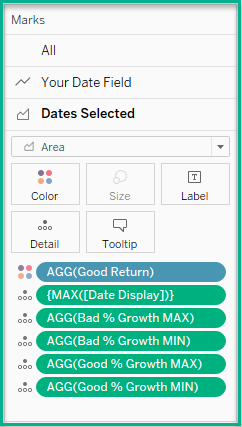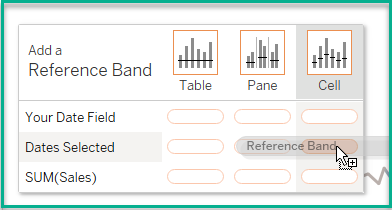top of page

# Area Chart in a Reference Band?

If you are new to Tableau you may not be aware of the Reference Lines feature. Tableau can add a stagnant line to your Line graph displaying an average value or target value if you prefer. Tableau can also add a Reference Band to your graph.

An example use case would be, what if you wanted to look back 30 days or more for your Sales or Profit metrics? What if you wanted a quick way to identify which Region, or Regions, were performing poorly for that time?

In the steps below I will review how to add a Reference Band for 30 (or more days) with a Parameter you can set. I will then go over adding an Area Chart to this Reference Band.I reverse engineered this tip from one of Will Perkin's Tableau Public Dashboards here. I changed some of the calculations and do not use all of the features he has in his dashboard.

The first thing I will do is create a Calculated field to mock up the Superstore data for my dashboard to include future dates. This will make the line graph appear to have projected sales.

I will do that with my calculation below;

Next I will create a Parameter for the Axis. This will adjust the axis of the line graph so it zooms in to see the values. It will reduce the number of months in the view.I will create another Parameter for the days;I put 50 to test but you can change this to 30.

Next we will create a series of calculations for our line graph;

Max Date Value Calc

today()

*This formula will used to display the end of our Reference Band in another calculation.

Min Date Value Calc

[Max Date Value Calc] - [Lookback Days]

*This formula will be used to display the beginning of our Reference Band in another calculation.

Dates Selected

IF [Your Date Field] >= [Min Date Value Calc]

AND [Your Date Field] <= [Max Date Value Calc]

AND [Min Date Value Calc] <> [Max Date Value Calc]

ELSEIF [Min Date Value Calc] = [Max Date Value Calc]

END

*This calculation will be used in a Dual axis with Your Date Field and will be used for the Area Chart display.

Date Range Display

IF [Axis Date Range] = "3 Months" then DATEADD('month',-3,[Max Date Value Calc]) < [Your Date Field]

ELSEIF [Axis Date Range]= "6 Months" then DATEADD('month',-6,[Max Date Value Calc]) < [Your Date Field]

ELSEIF [Axis Date Range]= '1 Year' then DATEADD('year',-1,[Max Date Value Calc]) <[Your Date Field]

END

*This calculation will help us use the Parameter we created for the axis dates.

Date Display

IIF([Min Date Value Calc]=[Max Date Value Calc],[Min Date Value Calc],NULL)

*This formula will help us for the Area chart, it will help us fill in any visual holes in the data.

Now I will take a break from Calculations and start to build the graph;

Next I add Dates Selected to the Columns shelf. I formatted both these fields as Exact Date.

I also add Region and Sum(Sales) to the Rows shelf.

I made the Dates Selected a Dual Axis with the Your Date Field. I also synchronized the axis and moved Your Date Field to be in front of Dates Selected.Next I changed the Dates Selected Marks card type to be an Area Chart.

Then I put the Date Range Display on the Filter Marks Card. I excluded the FALSE values.

I needed to create some more calculated fields to display how the last 30 days Sales performed. Did one Region do better than another?

Max Date Sales

{FIXED [Date Range Display], [Region] :

SUM( IIF({FIXED [Region] : MAX([Dates Selected])} = [Your Date Field], [Sales],0))}

*This Level of Detail (LOD) expression fixes the aggregation on Range and by the region. It also sums the Sales only by Region for the maximum date selected.

Min Date Sales

{FIXED [Date Range Display], [Region] :

SUM( IIF({FIXED [Region] : MIN([Dates Selected])} = [Your Date Field], [Sales],0))}

*Alternatively the LOD expression above does a similar thing to the previous one except it finds the minimum date selected.

Good Return

IF ([Min Date Value Calc]<= MIN([Dates Selected]) AND MAX([Dates Selected]) <= [Max Date Value Calc])

AND (((SUM([Max Date Sales]) - SUM([Min Date Sales])) /SUM ([Min Date Sales])) * 100) > 0 then 1

ELSEIF ([Min Date Value Calc]<= MIN([Dates Selected]) AND MAX([Dates Selected]) <= [Max Date Value Calc])

AND (((SUM([Max Date Sales]) - SUM([Min Date Sales])) /SUM ([Min Date Sales])) * 100) < 0 then -1

ELSE 0

END

*This formula identifies the Sales for the Region and range selected. It returns a 1 if the Sales % is greater than 0. It returns a negative 1 for the Sales % less than 0. All others are 0.

Next I placed Good Return on the Color card of Your Date Field Marks card. I also placed Region on the Detail.

I then added Good Return on the Color card of the Dates Selected Marks card. I also made it Discrete.I also changed the colors to dark burgundy for the -1 values. I made it lighter blue for the values of 1, using the Color Blind Color palette of Tableau since 20% of the population cannot see RED / AMBER / GREEN;I also needed to drag Date Display to Detail on the Dates Selected Area Chart Marks card. I had to edit this metric by right-clicking on it and selecting Edit in Shelf.

I changed this metric to read {MAX([Date Display])}. I also had to change it to the Exact Date.

Now I am back to creating some more Calculated Fields. This helped me with creating the Reference Band.

IF [Max Date Value Calc] <> [Min Date Value Calc]

AND Max([Date Range Display]) = TRUE

AND SIGN(ZN([Good Return]))= -1

THEN [Max Date Value Calc]

END

IF [Max Date Value Calc] <> [Min Date Value Calc]

AND Max([Date Range Display]) = TRUE

AND SIGN(ZN([Good Return]))= -1

THEN [Min Date Value Calc]

END

Good % Growth MAX

IF [Max Date Value Calc] <> [Min Date Value Calc]

AND SIGN(ZN([Good Return]))=1

then [MAX Date Value Calc]

END

Good % Growth MIN

IF [Max Date Value Calc] <> [Min Date Value Calc]

AND SIGN(ZN([Good Return]))=1

then [Min Date Value Calc]

END

*ZN formulas (used above) are great for returning 0 instead of NULL values.

I needed to put all four of these calculations on the Your Date Field and Dates Selected Marks Cards. I needed to also format them as Continuous.Then I went to the Analytics Data pane.

I selected Reference Band and moved it to the Cell option for Dates Selected.On the Edit Reference Line, Band or Box pop-up menu, I set up the band using the Bad % Growth MAX and MIN calculated fields as shown below;I picked a light pink for the back ground fill.

A red box should display for the 30 day lookback of the bad return.I needed to add a second Reference Band to highlight the good returns.

I repeated the steps above but filled out my Reference Band pop-up menu as below;This added a light blue background for the good metrics.This dashboard is located in my Tableau Public profile here all the way at the bottom of the dashboard.